EL10A TUTORIAL #2 PART 1   Saturday, 8 February 2003

1. A network is arranged as shown. Calculate the value of i by (a) Kirchoff's Laws (b) Superposition (c) Thevenin's Theorem (d) Norton's Theorem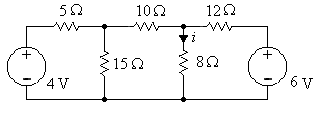2. Calculate the equivalent resistance between (a) A and B (b) A and N3. Using Thevenin's Theorem, replace the network to the left of terminals a-b by its equivalent and find i for the simplified circuit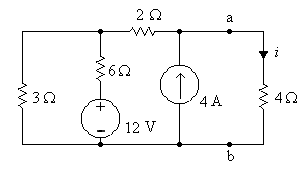4. Find i by replacing the network to the left of terminals a-b with it's Norton equivalent circuit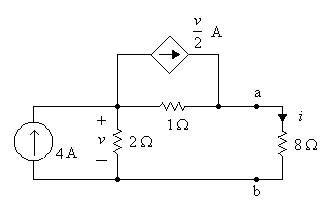5. Find v by replacing the network to the left of terminals a-b with it's Thevenin equivalent circuit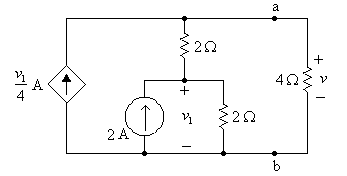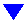PAGE TWO of your tutorial